Courses

# Test: Logarithm- 4

## 15 Questions MCQ Test Quantitative Aptitude (Quant) | Test: Logarithm- 4

Description
This mock test of Test: Logarithm- 4 for CAT helps you for every CAT entrance exam. This contains 15 Multiple Choice Questions for CAT Test: Logarithm- 4 (mcq) to study with solutions a complete question bank. The solved questions answers in this Test: Logarithm- 4 quiz give you a good mix of easy questions and tough questions. CAT students definitely take this Test: Logarithm- 4 exercise for a better result in the exam. You can find other Test: Logarithm- 4 extra questions, long questions & short questions for CAT on EduRev as well by searching above.
QUESTION: 1

### If log4 5 = a and log5 6 = b then what is the value of log3 2?

Solution: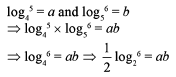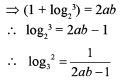QUESTION: 2

### If 2 [log (x + y) - log 5] = logx + logy, then what is the value of x2 + y2?

Solution: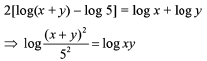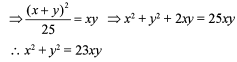QUESTION: 3

###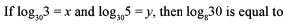Solution: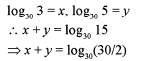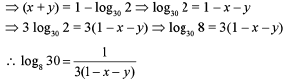QUESTION: 4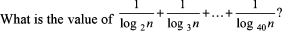Solution: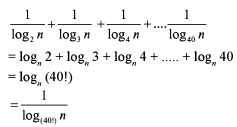QUESTION: 5

What is the value of  log32.log43.log54...log1615?

Solution: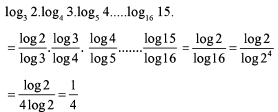QUESTION: 6

log 32700 = ?

Solution:

Log 32700 = log 3.27 + log 10000 = log 3.27 + 4

QUESTION: 7

If log102 = .301 find log10125.

Solution:

log10 125 = log10( 1000/8) = log. 1000 - 31og2 = 3 - 3 x 0.301 = 2.097

QUESTION: 8

log153375 x log41024 = ?

Solution:

log153375 x Log41024 = 3 log151 5 x 5 log4 4 = 3 x 5 = 15.

QUESTION: 9

log625 √5 = ?

Solution:

1/2 log625 5 = [1/(2 x 4)] log55 = 1/8.

QUESTION: 10

3 log 5 + 2 log 4 - log 2 = ?

Solution:

3 log 5 + 2 log 4 - log 2

= log 125 + log 16 - log 2

= log (125 x 16)/2

= log 1000 = 3.

QUESTION: 11

log (12 - x) = -1

Solution:

1/10 = 12 - x => x = 11.9

QUESTION: 12

log 2X = 3

Solution:

x log 2 = 3

log 2 = 3/x.
Therefore, x = 3/log 2

QUESTION: 13

5X = 10

Solution:

x = log510 = l/log105 = 1/log 5.

QUESTION: 14

find x If log x = log7.2 - log 2.4

Solution:

log x = log (7.2/2.4) = log 3 ⇒ x = 3

QUESTION: 15

Find x if logx =2 log 5 + 3 log 2

Solution:

log x = log 25 + log 8 = log (25 x 8 ) = log 200.